# What Math Is on the GMAT? Topics, Questions, and Review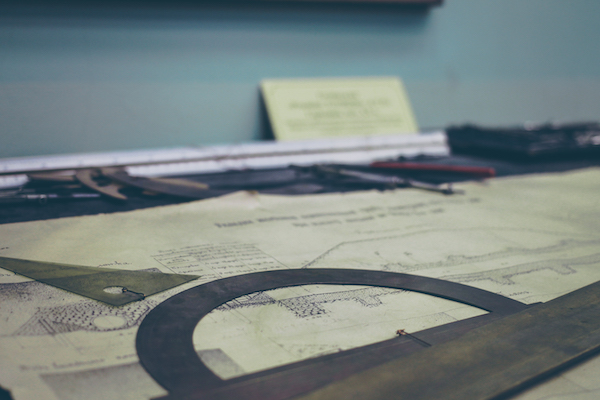The Quantitative section of the GMAT strikes fear into the hearts of many test takers who haven’t taken a math class in years. If you’re one of those test takers, you shouldn’t worry! You can totally handle the math in this section.

While the Quantitative section is challenging, it doesn’t test especially advanced concepts. This guide will show you what math is on the GMAT, along with key tips on how to prepare.

First, let’s discuss a general overview of GMAT math.

## GMAT Math Section: An Overview

The GMAT Quantitative section is your third section on the GMAT. You’ll take it after the 30-minute Analytical Writing Assessment, 30-minute Integrated Reasoning section, and an optional eight-minute break.

The Quantitative section is the first adaptive section on the GMAT, meaning that the questions change to match your ability level. You’ll start out with some medium level GMAT math questions, and the problems will get easier or harder depending on how you’re doing.

Not sure how or what to study? Confused by how to improve your score in the shortest time possible? We've created the only Online GMAT Prep Program that identifies your strengths and weaknesses, customizes a study plan, coaches you through lessons and quizzes, and adapts your study plan as you improve.

We believe PrepScholar GMAT is the best GMAT prep program available, especially if you find it hard to organize your study schedule and don't want to spend a ton of money on the other companies' one-size-fits-all study plans.The GMAT math section will continue to give you customized questions to get a more and more accurate measure of your skills. This adaptive format allows for comparable scores across different tests, plus it enhances security since no two tests look exactly the same.

You’ll get 31 GMAT math questions in 62 minutes, leaving you with approximately two minutes per question. Unlike the Integrated Reasoning section, you won’t have access to a calculator. You will get some note boards and markers to write out your work.

While you’ll need to know a variety of math concepts for this section, you’ll only see two question types. Read on to learn what they are.

## GMAT Math Questions: 2 Types

There are just two types of Quantitative questions, and they’re interspersed throughout the section in random order. The two types are problem solving questions and data sufficiency questions.

Typically, about two-thirds of GMAT math questions will be problem solving and one-third will be data sufficiency. However, this proportion could change depending on how many experimental questions you get of each type.

Problem solving questions are just like any other typical question you’d get on a math test. They may ask you to solve an equation, figure out a word problem, or answer questions on a graph.

Each question has five answer choices, and there’s only one right answer for each. Where these questions do get tricky is when they require multiple steps or abstract thinking.

Here’s one example of a problem solving question on the Quantitative section of the GMAT.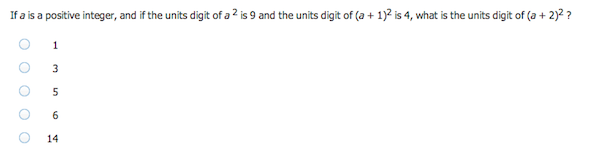Answer: A

The second type of Quantitative question, data sufficiency problems, are more unusual. These questions present you with a math problem followed by two pieces of information. You don’t have to solve the problem, but rather indicate whether one or both statements give you “sufficient data.”

Here’s an example of a GMAT math data sufficiency problem.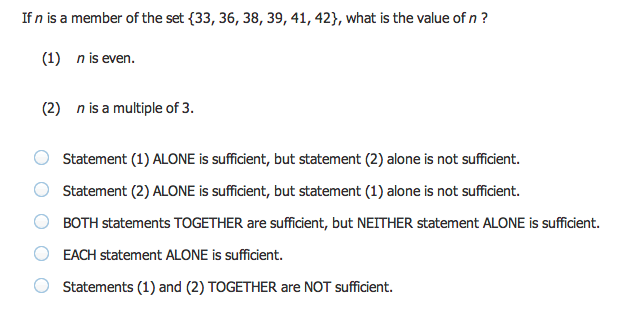The answer choices for data sufficiency questions are always the same as the ones you see above. You don’t need to solve the initial problem. You just need to know if you could solve the problem based on the given information.

While there are only two question types in the Quantitative section, there are quite a few more concepts that show up. Below, you’ll find an overview of the tested GMAT math concepts, along with sample problems for each one.There are just two types of GMAT math questions, problem solving and data sufficiency.

## What Math Is on the GMAT Quantitative Section?

As you read above, the Quantitative section doesn’t require you to be a mathematician. Your GMAT math review won’t involve advanced calculus or trigonometry. In fact, the GMAT math topics don’t get much more advanced than high school-level algebra.

The main challenge for a lot of people, then, is reviewing concepts that they studied in the past but haven’t used in a long time. The main skills you need to answer both question types involve arithmetic, algebra, geometry, and word problems.

Let’s take a closer look at the topics within each of these skill areas as outlined by the official GMAT Prep Software, starting with arithmetic.

### GMAT Math Topics in Arithmetic

You’ll get a variety of problems that require skills in arithmetic. You shouldn’t have to do particularly advanced calculations, since you won’t have use of a calculator, but the questions may require some strategic problem solving and complex thinking.

Want to improve your GMAT score by 60 points?

We have the industry's leading GMAT prep program. Built by Harvard, MIT, Stanford, and Wharton alumni and GMAT 99th percentile scorers, the program learns your strengths and weaknesses and customizes a curriculum so you get the most effective prep possible.For arithmetic math in the Quantitative section, you should be familiar with

• Properties of integers
• Fractions
• Decimals
• Real numbers
• Ratio and proportion
• Percents
• Powers and roots of numbers
• Descriptive statistics
• Sets
• Counting methods
• Discrete probability

The problem below, for example, is a problem solving GMAT math question that involves fractions and percents.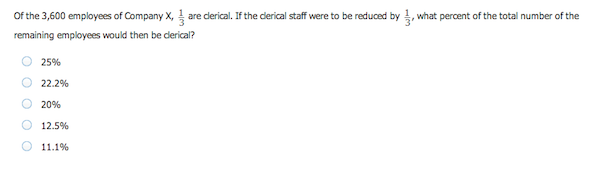Answer: A

This second sample problem is a data sufficiency question that calls on your arithmetic and logic skills.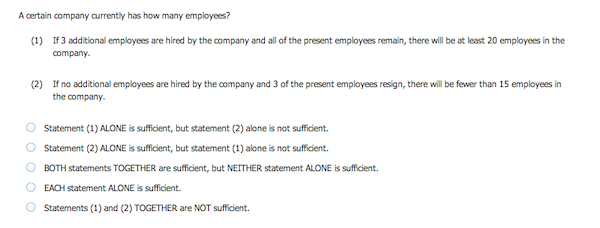Get ready to count your fingers and toes. There’s a lot of arithmetic in the GMAT math section.

### GMAT Math Topics in Algebra

In addition to arithmetic, another major skill area is algebra. As part of your GMAT math review, you definitely want to get work with algebraic expressions and solve for variables. You should be comfortable with

• Variables and algebraic expressions
• Manipulating algebraic expressions
• Equations
• Solving linear equations with one unknown
• Solving two linear equations with two unknowns
• Solving equations by factoring
• Solving quadratic equations
• Exponents
• Inequalities
• Absolute value
• Functions

The sample question below is a typical problem solving question that involves an algebraic expression with two variables and an exponent.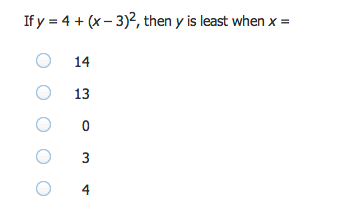Answer: D

Data sufficiency questions may also call on your algebra skills, like in the practice question comparing two variables below.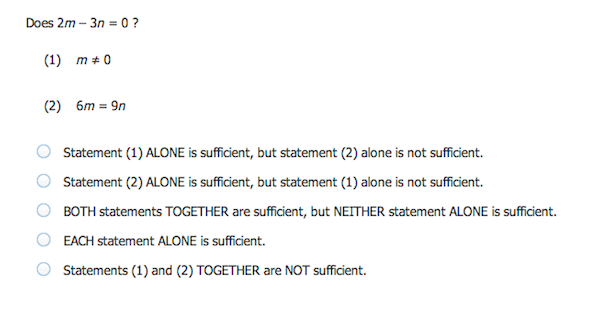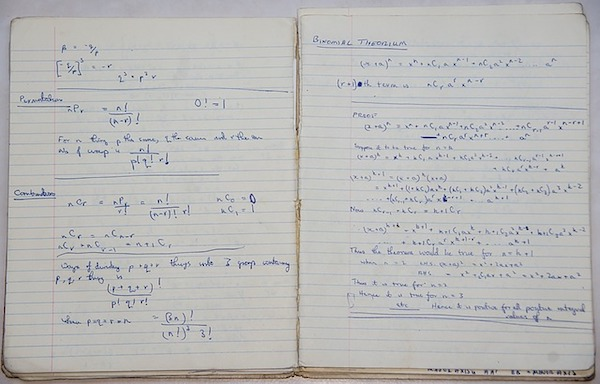Hopefully, you kept your high school algebra notes. Didn’t think they’d come in handy someday, did you?

### GMAT Math Topics in Geometry

You won’t find quite as many questions on geometry as on arithmetic and algebra, but they definitely still come up. To prepare, you should study the following concepts:

• Lines and angles
• Polygons (convex)
• Triangles
• Circles
• Rectangular solids and cylinders
• Coordinate geometry

This problem solving question, for instance, asks about properties of triangles.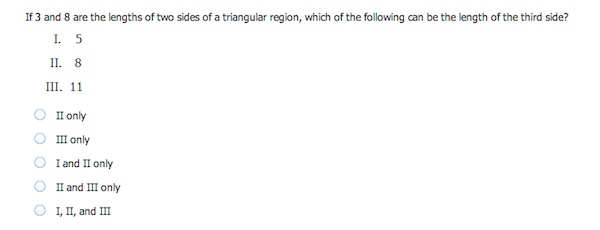This data sufficiency question requires you to have some understanding of angles and how they relate to one another.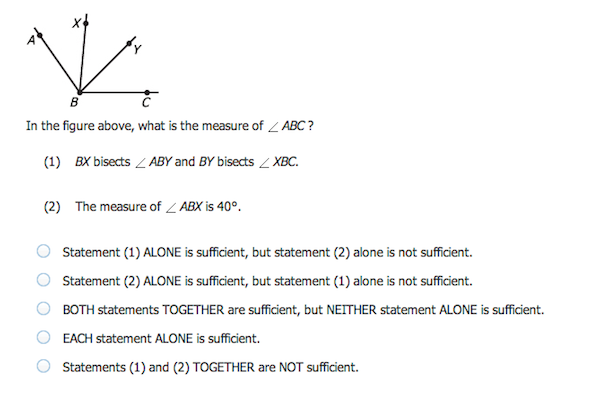Answer: CParallel lines have so much in common. It’s too bad they’ll never meet.

### GMAT Word Problems

Word problems may call on arithmetic, algebra, or geometry skills, plus they require careful reading to identify exactly what the problem is asking you to do.

Want to Identify YOUR GMAT Strengths and Weaknesses?

Our proprietary GMAT Diagnostic Assessment creates a customized study plan for you that takes you from registration all the way to test day! It is included with every account and proven to significantly maximize your score.

Get your personalized assessment as part of your 5 day risk-free trial now:You might be asked to calculate simple interest or compound interest, calculate rate, or measure profits. You also may have to work with data from a table, line chart, bar graph, scatter plot, or pie graph. Finally, a question may ask you to analyze sets, as presented in Venn diagrams, or analyze probability.

These are some typical concepts you need to understand to solve GMAT word problems.

• Rate
• Work (these questions typically show the rates at which a person and a machine work alone, and you’ll have to compute the rate at which they work together, or vice versa)
• Mixture (in these problems, substances with different characteristics are combined, and you’ll need to determine characteristics of the resulting mixture)
• Interest
• Discount
• Profit
• Sets
• Geometry
• Measurement
• Data interpretation

This problem solving practice question asks you to calculate how fast the Earth travels around the sun. You need to convert miles per second into miles per hour.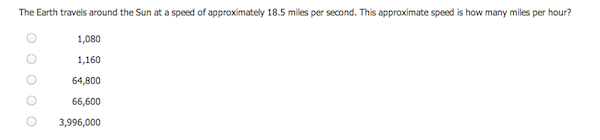Answer: D

This data sufficiency question is a word problem that calls on your arithmetic and logic skills.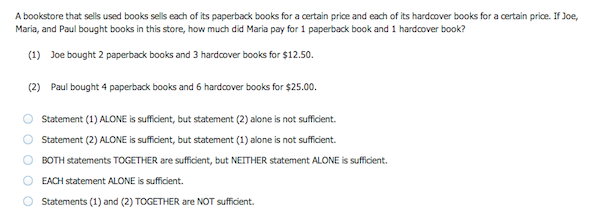Answer: E

Knowing what math is on the GMAT is an important first step, but what else can you do to prepare for the Quantitative section? Read on for six key tips on getting ready for GMAT math.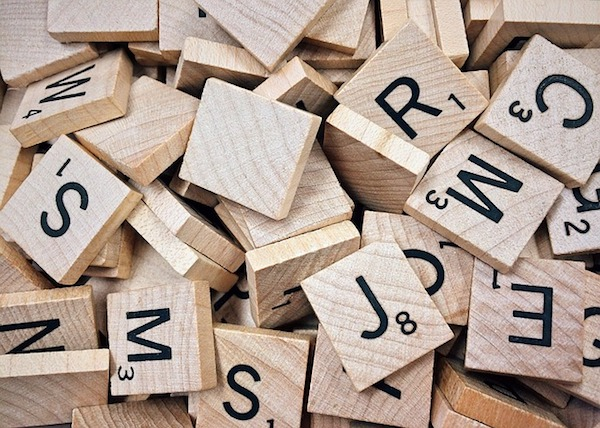Words and numbers come together in GMAT word problems.

## How to Review Math for the GMAT: 6 Key Tips

How can you get ready for the problem solving and data sufficiency questions on the Quantitative section? What can you do to review concepts that you may not have seen since high school?

Read on for six tips to guide your GMAT math review.

### #1: Review the Fundamentals

As you read above, GMAT math doesn’t involve particularly advanced concepts. You won’t need calculus, trigonometry, or any college-level mathematics.

Your first step in preparing, then, should be to review fundamental math concepts. You need to have a solid foundation of key concepts in arithmetic, algebra, and geometry, plus a firm grasp of logic and analysis that will help you solve word problems.

Make sure your study materials offer a thorough review of all tested concepts, and take time to work through the lessons.

### #2: Drill With Realistic Practice Questions

As you review key concepts, you should reinforce your understanding with GMAT math practice problems. Even though the math in the Quantitative section isn’t particularly advanced, the questions are still challenging.

They often require complex thinking and multiple steps. Even if you know how to calculate the area of a right triangle or solve a quadratic equation, you need to make sure you can apply that knowledge to GMAT math questions.

By drilling with realistic practice questions, you’ll learn how to apply your math knowledge to the GMAT. The best practice questions come from the official test makers, as on the GMAT Prep Software. Third party test prep companies, like Kaplan and Manhattan Prep, also offer useful practice questions and tests.

As you answer the questions, take notes on anything that confuses or trips you up. Take time to read answer explanations and find the source of your confusion. By understanding your mistakes, you can take specific steps to fix them.

### #3: Take Timed Practice Tests

After you’ve done initial concept review and tried out some practice problems, you should set aside time to take a timed GMAT practice test. Find a quiet place, take a practice test with a computer adaptive format, and give yourself 62 minutes to complete the section.

After you’ve finished the practice test, take time to review any questions that you got wrong or felt unsure about. Try to locate the source of your error.

Were you running out of time? Did you misunderstand what a question was asking you to do? Did you make an arithmetic mistake? Were you unfamiliar with a concept? Did you simply make a careless error?

By figuring out where you went wrong, you can take the right steps to fix it for next time. The practice test will not only help you figure out what concepts you need to review, but it will also give you feedback on your pacing. Doing well on the Quantitative section requires you to work quickly and efficiently, and taking practice tests will help you improve your test taking rhythm.

### #4: Memorize Data Sufficiency Answer Choices

Data sufficiency questions are weird; there’s no doubt about it. You won’t really see questions like them anywhere but on the GMAT.

To minimize confusion, you should familiarize yourself with the answer choices before test day. You’ll always see the same five answer choices that ask whether statement 1, statement 2, both, or neither give you sufficient information to solve the problem.

Remember that you don’t need to solve these problems, but rather indicate whether you have enough information to solve them. Memorize the answer choices before test day so you know exactly what you’re looking for in each data sufficiency problem and don’t have to waste time reading through or trying to differentiate between the answer choices.Before you take the GMAT, you should know the answers to data sufficiency questions like the back of your (perfectly manicured) hand.

### #5: Write Out Your Work

As goes the mantra of high school math teachers everywhere, make sure to show your work. You can’t use a calculator in the Quantitative section, and mental math will only get you so far.

You’ll get note boards and markers in the testing center to write out your work. You should definitely use them to work through the challenging, often multi-step problems.

Try to be neat and organized so you don’t run out of room or make a careless error. If your answer doesn’t match any of the answer choices, then you may need to comb through your work to find the calculation mistake. Try to keep everything legible to make things easier on yourself.

These note boards won’t be graded and are entirely for your own use. You can use them on any of the GMAT sections, but they’re especially helpful when solving math problems.

### #6: Practice, Practice, and Practice Some More

As a skill like any other, GMAT math demands a lot of dedicated practice. Whether or not you consider yourself a “math person,” you should set aside time to review concepts, answer sample questions, and take practice tests.

Not only will you sharpen your math skills, but you’ll also become a more efficient test-taker who can handle answering 31 questions in 62 minutes. Don’t get discouraged, but know that you can improve with effort, time, and a customized study plan.

## GMAT Math Questions: Final Points

To prepare for the Quantitative section of the GMAT, you should focus on fundamental concepts of arithmetic, algebra, and geometry, plus you need to prepare for word problems. You’ll answer two types of questions, the straightforward problem solving questions and the more unusual data sufficiency questions.

After months of GMAT math review, you can go into the test familiar with these question types. You can hone your time management skills by taking timed practice tests. You’ll also find that the math section gets more and more manageable as you answer lots of GMAT practice questions.

You don’t need to be a math person to do well on the GMAT Quantitative section, but you do need to spend time preparing. With enough effort, you can sharpen skills that you first developed in high school and get yourself ready to conquer GMAT math.

## What’s Next?

Now you’re an expert in GMAT math, but do you know what the rest of the exam looks like? Check out our guide to the full GMAT structure and format, along with examples of each question type across the test.

Do you know how the GMAT is scored? Check out our complete guide to GMAT scoring, and then head to this article to see how section and total scores correspond to percentiles.

The GMAT is a daunting test, but how hard is it, really? This article tackles that complicated question to show you just how challenging you can expect the GMAT to be.